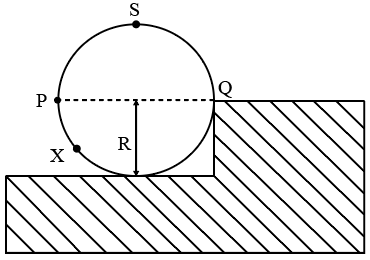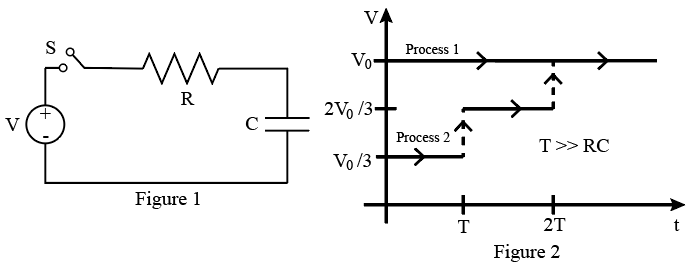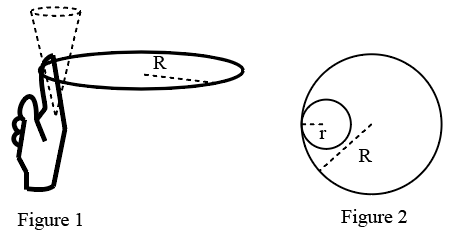Instructions

For the following questions answer them individually

Question 11

Question 12

Question 13

Question 14

# A wheel of radius R and mass M is placed at the bottom of a fixed step of height R as shown in the figure. A constant force is continuously applied on the surface of the wheel so that it just climbs the step without slipping. Consider the torque $$\tau$$ about an axis normal to the plane of the paper passing through the point Q. Which of the following options is/are correct?Instructions

Consider a simple 𝑅𝐶 circuit as shown in Figure 1.

Process 1: In the circuit the switch 𝑆 is closed at t = 0 and the capacitor is fully charged to voltage $$V_0$$ (i.e., charging continues for time $$T \gg RC$$). In the process some dissipation $$(E_D)$$ occurs across the resistance 𝑅. The amount of energy finally stored in the fully charged capacitor is $$E_c$$.

Process 2: In a different process the voltage is first set to $$\frac{V_0}{3}$$ and maintained for a charging time $$T \gg RC$$. Then the voltage is raised to $$\frac{2 V_0}{3}$$ without discharging the capacitor and again maintained for a time $$T \gg RC$$. The process is repeated one more time by raising the voltage
to $$V_0$$ and the capacitor is charged to the same final voltage $$V_0$$ as in Process 1.

These two processes are depicted in Figure 2.Question 15

Question 16

# In Process 2, total energy dissipated across the resistance $$E_{D}$$ is:

Instructions

One twirls a circular ring (of mass M and radius R) near the tip of one’s finger as shown in Figure 1. In the process the finger never loses contact with the inner rim of the ring. The finger traces out the surface of a cone, shown by the dotted line. The radius of the path traced out by the point where the ring and the finger is in contact is r. The finger rotates with an angular velocity $$\omega_{0}$$. The rotating ring rolls without slipping on the outside of a smaller circle described by the point where the ring and the finger is in contact (Figure 2). The coefficient of friction between the ring and the finger is $$\mu$$ and the acceleration due to gravity is gQuestion 17

Question 18

# The minimum value of $$\mu$$ below which the ring will drop down is

Instructions

For the following questions answer them individually

Question 19

Question 20

OR